# GSEB Solutions Class 8 Maths Chapter 13 Direct and Inverse Proportions Intex Questions

Gujarat Board GSEB Textbook Solutions Class 8 Maths Chapter 13 Direct and Inverse Proportions Intex  Questions and Answers.

## Gujarat Board Textbook Solutions Class 8 Maths Chapter 13 Direct and Inverse Proportions

Try These (Page 204)

Question 1.
Observe the following tables and find if x and y are directly proportional?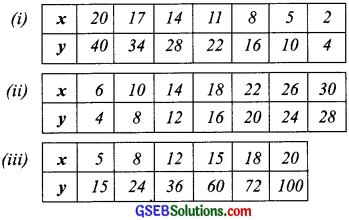Solution:
(i)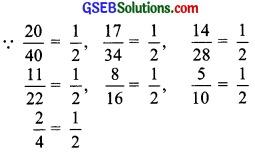i.e; Each ratio is the same.
∴ x and y are directly proportional

(ii)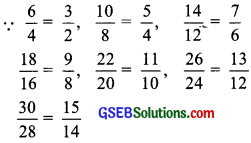i.e; All the ratios are not the same.
∴ x and y are not directly proportional

(iii) ∵ $$\frac{5}{15}$$ = $$\frac{1}{3}$$,
$$\frac{8}{24}$$ = $$\frac{1}{3}$$,
$$\frac{12}{36}$$ = $$\frac{1}{3}$$
$$\frac{15}{60}$$ = $$\frac{1}{4}$$,
$$\frac{18}{72}$$ = $$\frac{1}{4}$$, $$\frac{20}{100}$$ = $$\frac{1}{5}$$
i.e; All the ratios are not the same.
∴ x and y are not directly proportionalQuestion 2.
Principal = ₹ 1000, Rate = 8% per annum. Fill in the following table and find which type of interest (simple or compound) cluinge is in direct proportion with time period?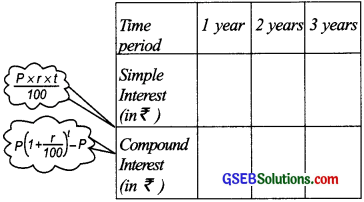Solution:
Case of simple interest [P = ₹ 1000, r = 8% p.a.]∵ In each case the ratio $$\frac{SI}{T}$$ is the same.
∴ The simple interest changes in direct proportion with time period.
Case of Compound Interest [P = ₹ 1000, r = 8% p.a.]∵ $$\frac{CI}{T}$$ is not the same in each case.
∴ The compound interest does not change in direct proportion with time period.Try These (Page 208)

Question 1.
On a squared paper, draw five squares of dfferent sides. Write the following information in a tabular form?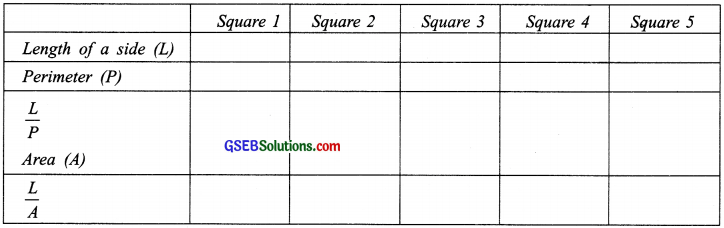Find whether the length of a side is in direct proportion to:
(a) The perimeter of the square
(b) The area of the square
Solution:
We draw the following squares I, II, III, IV and V having the length of side as 1 cm, 2 cm, 3 cm, 4 cm and 5 cm respectively.
Now, we have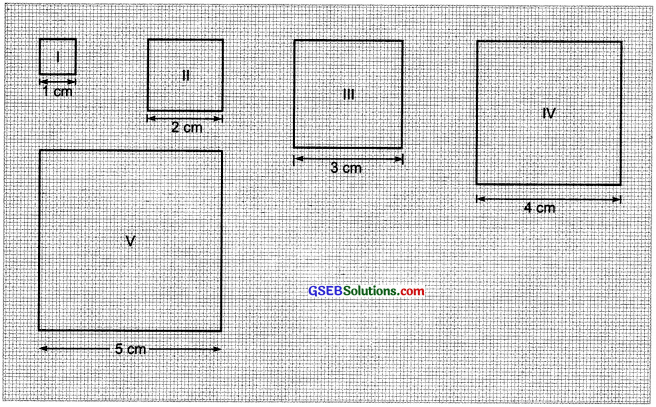Obviously:
(a) The length of a side (L) and the perimeter of the square (P) are in the direct proportion.
(b) But the length of a square (L) and the area of the square (A) are not in the direct proportion.Try These (Page 211)

Question 1.
Observe the following tables and find which pair of variables (here x and y) are in inverse proportion?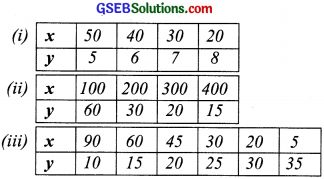Solution:
(i) ∵ x1 = 50 and y1 = 5
⇒ x1y1 = 50 × 5 = 250
x2 = 40 and y2 = 6
⇒ x2y2 = 40 × 6 = 240
x3 = 30 and y3 = 7
⇒ x3y3 = 30 × 7 = 210
x4 = 20 and y4 = 8
⇒ x4y4 = 20 × 8 = 160
Also 250 ≠ 240 ≠ 210 ≠ 160 or
x1y1 ≠ x2y2 ≠ x3y3 ≠ x4y4
∴ x and y are not in inverse proportion.

(ii)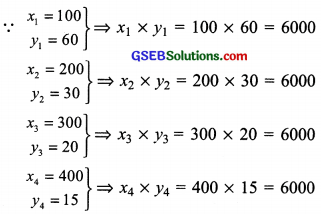and x1y1 = x2y2 = x3y3 = x4y4
∴ x and y are not in inverse proportion.

(iii)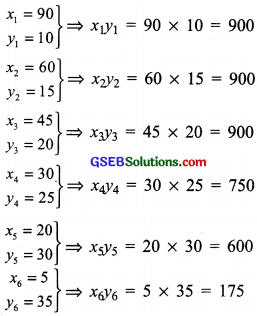and x1y1 = x2y2 = x3y3 ≠ x4y4 ≠ x5y6 ≠ x6y6
∴ x and y are not in inverse proportion.

Try These (Page 215)

Question 1.
Take a sheet of paper. Fold it as shown in the figure. Count the number of parts and the area of a part in each case?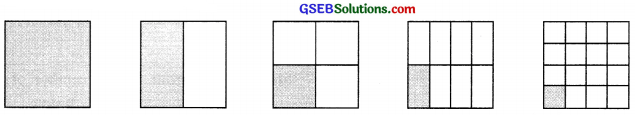Tabulate your observations and discuss with your friends. Is it a case inverse proportion? Why?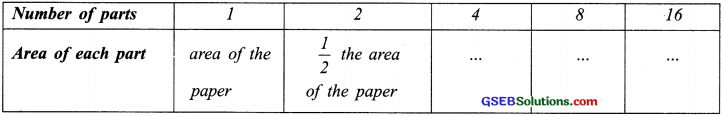Solution: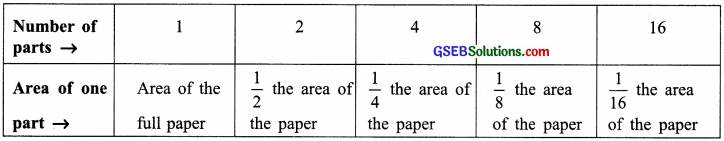Here, more the number of parts, lesser is the area of each part.
∴ It is a case of “inverse proportion”.Question 2.
Take a few containers of different sizes with circular bases. Fill the same amount of water in each container Note the diameter of each container and the respective height at which the water level stands. Tabulate your observations. Is it a case of inverse proportion?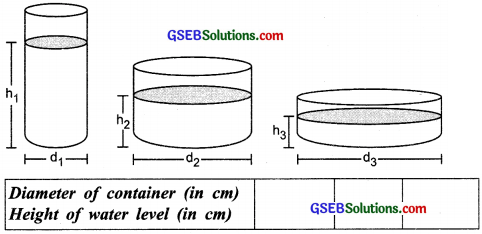Solution: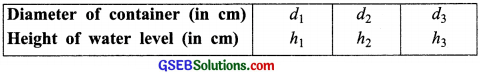Here, lesser the diameter, more is the level of water in the container.
∴ It is a case of inverse proportion.